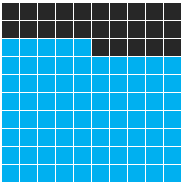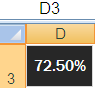# Create Amazing Percentage Chart in ExcelToday I am going to cover how to create a percentage chart in excel.

## Percentage Chart

It allows you to create your own chart by entering percentage.

## Steps

1. Select 10 Rows and 10 Columns (10 X 10 Matrix). Let's say G7:P16.

2. Shade to cells by filling them with black color and apply border to the cells (All Borders).

.

3. Enter a percentage value in cell D3.4. Select G7:P16 cells and apply conditional formating using a formula.

Go to Conditional Formating >> Select "New Rule" >> Select "Use a formula to determine which cells to format"

And put the following formula in the formula box.

=((ROW(\$P\$16)-ROW())*10+COLUMN()-COLUMN(\$A\$1)+1)<=ROUND(\$D\$3*10^2,0)
ROW(P16) => Last row of your matrix. It returns 16.

COLUMN(A1) => First column of a sheet. It returns 1. It will always be the same no matter matrix is placed.

D3 => Cell in which your percentage value is placed

### Final Step

After pasting the above formula, click on Format button that appears just below the formula box >> Select Fill >> Select Light Blue color >> OKRelated Posts
Share